# Finite State Accounting Improves Strategy Evaluation

|
Includes: IWM, SPY, XLE, XLV, XRT
by: Josef Friedman
Summary

FSM - Finite State Machine concepts offer powerful technical insights into stock performance.

A Finite State Accountant - FSA - improves insight during strategy evaluation and development.

A two state FSA prototype is proposed and demonstrated.

## Introduction

Strategies are evaluated by analyzing past performance with historical price data. Current evaluation techniques are questionable because they simply confirm or contradict the biases of the strategy developer.

Ideally, an evaluation should provide additional insight by systematically considering other possibilities. This article discusses and demonstrating a methodology to accomplish this.

## Finite State Machines

It was a revelation when I first read about Finite State Machines in relation to algorithmic investing strategies recently.

“… a mathematical model of computation. It is an abstract machine that can be in exactly one of a finite number of states at any given time. The FSM can change from one state to another in response to some external inputs; the change from one state to another is called a transition. An FSM is defined by a list of its states, its initial state, and the conditions for each transition.”

The cool name might make the concept appear more complicated than it is; a light switch or turnstile are two examples – not exactly rocket science or even arithmetic.

I was introduced to this concept in The Ultimate Algorithmic Trading System Toolbox by George Pruitt. This book is a good introduction to the discipline and deserves its five star rating at Amazon.

My two prior SeekingAlpha articles on simple moving average strategies dealt with volatility and performance. These were written before I read George’s book. The FSM design concept is present in those articles; my recent research develops this further. This is my interpretation of the FSM concept, and I believe it is original.

## Basic Testing Framework

At the risk of causing the reader’s eyes to glaze over, let me discuss the testing framework briefly.

## Download and Calculating

An example of basic weekly downloaded price data for SPY is shown below: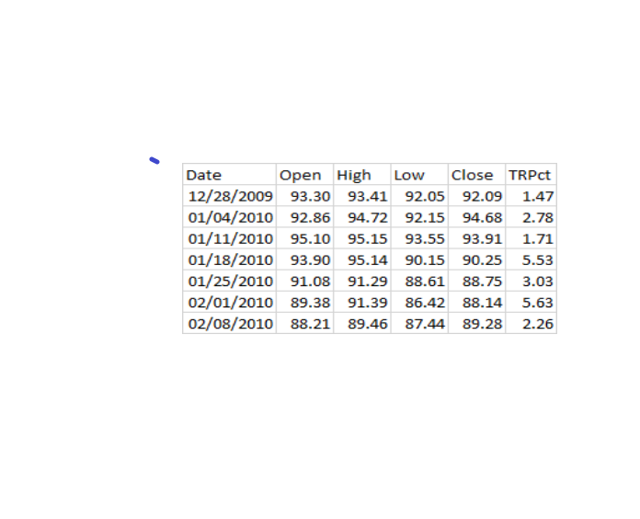Created by author using source data from Tiingo

The TRPct column is the period’s True Range Percent. The true range is the difference between the period’s High and Low prices unless the previous close is outside of that range, in which case it replaces either the high or low in the calculation. Once the true range is calculated; the VBA code for TRPct is:

TRPct = TrueRngX / CloseX * 100.

Note: Manually calculating this based on the numbers shown will have small differences to those shown here because of rounding. This is an important measure of volatility.

All the other indicators that I plan to look at are calculated during the data download.

Arguably, the two most important technical indicators of price action are the average price and the rate of change. Moving averages for the periods shown above are presented like this: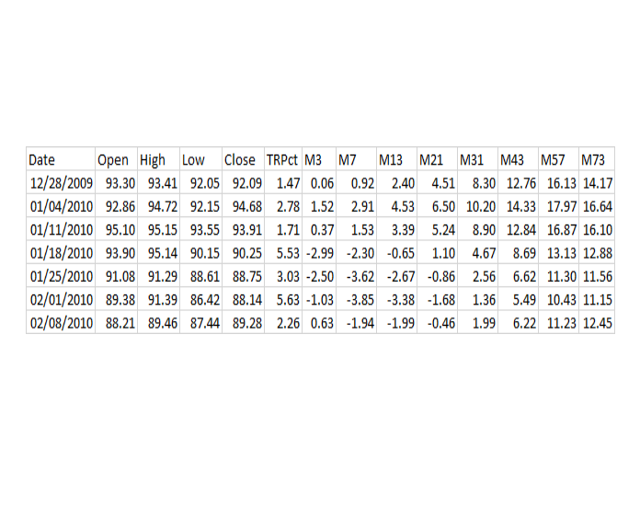Created by author using source data from Tiingo

“M” stands for moving average in the columns, and the number following it is the period length. For example, M3 is the 3 week moving average expressed as a percentage of the close price.

The moving averages used are calculated on what is generally known as the weighted price. (Open + High + Low + Close) * .25

During the download, an array of moving averages is built and the final numbers are calculated with the VBA code:

Worksheets(Symbol).Cells(x1, xw + 6) = (CloseX - Mx(xw)) / CloseX * 100

This conversion simplifies the analysis as it can be determined whether the current price is above or below a given average by simply checking if the number is positive or negative. Another major advantage is that it normalizes the numbers.

As part of my research, I decided to examine the numerical sequence shown; which goes, 1,3,7,13,21… The numbers are derived by adding consecutive even numbers. The selected numbers seem better than Fibonacci numbers for this application, as this sequence avoids the two dubious fib numbers 5 and 8, replacing them with the excellent number 7. Surprisingly, this sequence has no official name; my humble suggestion is Friedmanacci numbers.

Rate of change numbers are also calculated for the same eight number sequence, in addition to the one day rate of change which I refer to as Roc1. With an FSM model, we are also keenly interested in a concept called Roc0. This is the rate of change on the day of a state transition. The state transition happens at the close of a period, in this case, end of the week. State retains the previous week’s state until the close. This can be referred to as day 0, hence Roc0.

## Benefits of the Framework

R3 through R73 will not be part of this discussion. However, it is worth mentioning that they share the same key properties as the moving average numbers, they are both percentages that can be either positive or negative.

State transitions between any of the two sets of eight numbers can be examined by simply providing column numbers. For example, one could say look at columns 9, 11, and 16 and report on what is going on in the eight possible states without any need to define the strategy further.

This separates the details of the strategy from the analysis.

## Analyzing the States

The historical price data is run through a Finite State Accountant. The FSA is a very simple VBA routine; the logic does not buy or sell, it accounts for state specific data. A sample is below.

Created by author using source data from Tiingo

I spent many hours trying to figure out how to avoid adding the Win column.

Profit is the difference between the starting and ending price of the state multiplied by the number of shares the base amount can buy. The FSA virtually invests a base amount of \$100,000 in SPY at whatever state it is in at the beginning of the analysis. The position is retained through state changes.

Typically, back testing applications use a fixed amount (shares or dollars) to invest for each trade. The FSA can be set up to do that, but the way it is done here also has excellent points. .

The advantage to keeping a running total is that holding through all state transitions will give the buy and hold profit as the total profit. The other advantage, and I should admit this is accidental, is that a very clear picture of the merits of each state is presented.

The FSA goes through the price data and writes a state description summary at the state transition, to a different spreadsheet. This contains data that I consider important. This version of the FSA looks at over and under zero state transitions of a single column which is supplied to the routine as a variable.

The FSA can analyze data from any time period without changing the routine as long as the data is in the expected format. Other critical design elements include the spreadsheet and state naming conventions.

A single data element with the properties we are discussing can be divided into two states: above or below zero (or some other number). The element can also be divided into 2 additional states based on whether the value is above or below the previous period's value. The FSA logic does not have to be changed based on the number of elements or possible states other than defining state conditions and element location. It can also analyze multiple securities without requiring manual intervention.

## Two State Structure

The original idea that inspired my articles was presented as “buy the S&P 500 when the price is above the 200 day simple moving average and sell when the price drops below”. This strategy has been consistently profitable since further back than most people care to look, but is not very good for reasons I outlined in my previous articles.

This strategy was proposed by Logan Kane in his fascinating, albeit flawed article which inspired my two previous replies.

There are semantic issues with the strategy definition that inhibit proper evaluation of it. The proposal implicitly divides the market into two states:

1. Price is above the given average
2. Price is not above the given average

The idea of buying and selling on state transitions is totally premature from an FSM analytical perspective at this point. Instead, we are interested in the market performance characteristics in all the defined states.

## Pivot Tables

Six months ago I really hoped that I could get through life without learning pivot tables. It takes some practice and the particular situation we are discussing here requires using VBA. I wish I could say it took me less than 100 hours to get to my modest level of knowledge.

Here is a pivot table showing the results of buying (and holding) SPY from 1/4/2010 through 7/10/2019 through a two state 43 week moving average lens. 43 weeks is close to 200 days.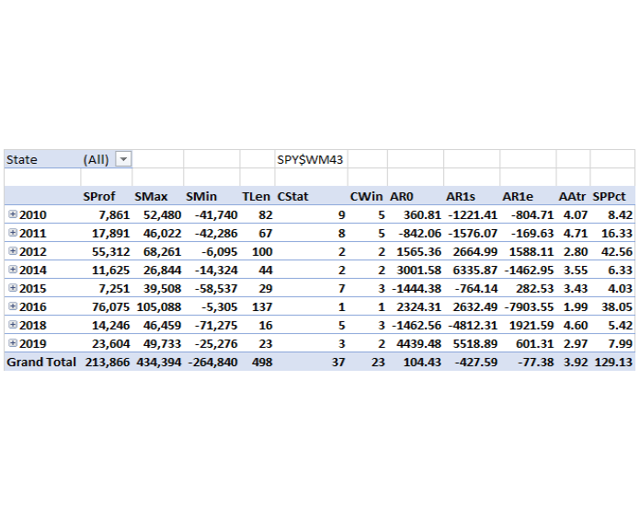Created by author using source data from Tiingo

I have to admit to cherry picking the dates of course, but there really isn't any possible date selection (from date n to the present) where being long only above the 43 week moving average has much attractiveness.

The Column SProf shows the net results by year. The grand total of 213,866 is the amount of profit one would have on a \$100,000 investment in SPY. The \$100,000 initial investment would now be worth \$313,866.

TLen is the number of weeks for state changes. The study is looking at 498 weeks of data. CStat is the number of state changes. The number of CStat changes that were profitable is in the column CWin.

Let us look at performance in the Above state.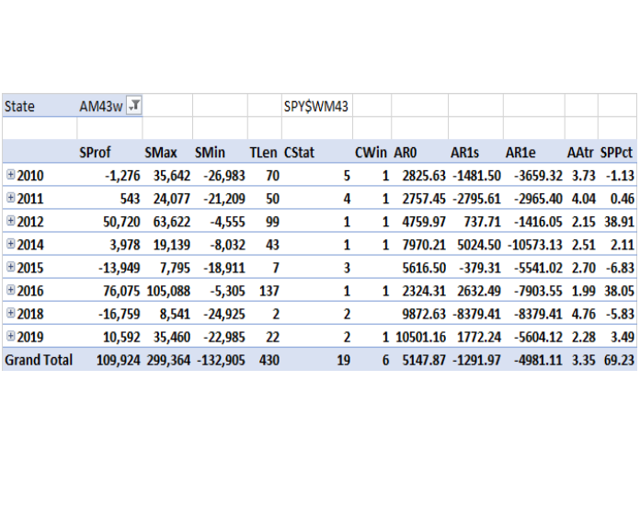Created by author using source data from Tiingo

Note the poor winning percentage, less than 33%. A lot of the dubious percentage is in 2010 through 2011 which suggests a period of technical adjustment from the financial crisis. We can't complain about the profit though.

2015 was difficult for AM43w. Note that the AM43w state had 3 losing years out of 9.5. That is misleading though, because profit made in the first nine months of 2018 is accounted for in the AM43w state that lasted for 137 weeks starting in 2016. Note how the spectacular profit in 2016-2017 is sandwiched between two difficult years.

The length of time in the above state is notable, 430 weeks out of 498 or 86% of the time. That number is over 10% above what I'd expect to see in a longer data sampling. Most of the profit was made during the above state, not much more though.

SMax is the sum of the maximum unrealized profit during the state. Note the total SMax is approaching 3 times as high as the Profit number. That implies inefficiency. The SMin number looks more or less normal to me.

AR0 is a major issue with an AM43w strategy. AR0 is the average amount of profit (or loss) forgone by not being long the week before the transition. There were 19 state transitions (from below to above) and the above state lost an average of over \$5000 on every one of them. A positive number is bad for AR0. This report is easier to understand if fixed investment amounts are used as then AR0, AR1s, and AR1e would be percentages. AR0 is about 3% on average.

AR1s is the average of Roc1 for the first week (period) of the state - "s" stands for start. Note that it is negative and not by a trivial amount.

SMax, AR1e and AR0 are the three major issues. AR1e is the average rate of change on the week that the state transition happens (e stands for end). It is almost as bad as AR0 in the table. These are structural issues with buying momentum. It will be present in most simple 2 state above to below transitions regardless of the length of the period measured. It will be less noticeable on shorter time periods and larger on longer.

Let us see how SPY performed when it transitioned to Below M43w.

Created by author using source data from Tiingo

The BM43w state lost money on a single trade in 2015 but had a profit on the other 17 state changes during the almost 10 year period.. Below states typically have better winning percentages, while above states make a little more money.

Note AAtr is 4.51 for BM43w but only 3.34 during AM434w. This is an average of the true range as discussed above and the average length is the length of the state in periods (TLen). This confirms a rise in volatility in Below situations. A below condition on any of the Friedmanacci moving averages will have a higher average true range than the above state, and the levels vary in proportion to the length of the average.

Total TLen for BM43w is 68 weeks, and you double your money. With AM43w it took 440 weeks to double.

Many aspects of action during below states are the opposite of above. Above does best with long TLen and fewer state changes. Below is best with short TLen and multiple state changes.

AR0 and AR1e confirm that the money squandered by the above state during transitions is picked up as a gain by the below state.

I was originally going to put examples of fixed amount investment results here but decided not to. Changing the FSA to fixed amount is trivial in any case. BaseAmount starts off as \$100,000, at a state transition the following lines of code are run:

BaseAmtX = (BaseAmount / BuyPrice * SellPrice)

BaseAmount = BaseAmtX

To change to a fixed amount, this code can simply be bypassed or commented out.

It is difficult to imagine someone who has seen this analysis deciding to mechanically sell SPY at the 43 week moving average.

## Monitoring a Portfolio

The FSA can process multiple securities at a time. An example is below: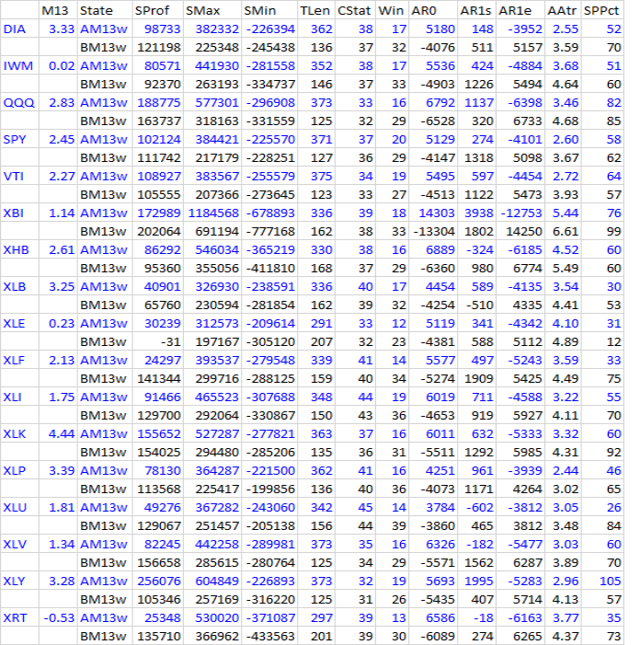Created by author using source data from Tiingo

This summarizes the major ETFs by activity above and below the 13 week moving average from the beginning of 2010. Note how every ETF except XLE (which was the worst performer) have substantial profits during the 10 year period. Note also how the data relationships mentioned in the previous section carry over to the other securities and time frames.

Note the higher winning percent for BM13w compared to AM13w.

The current M13w level for each security is next to the symbol. Attention should be paid to instruments near their transition states. Selling (if that is part of the plan) should probably be done somewhere in the area between SMax and SProf. Obviously further research is needed to identify reasonable points, probably mean reversion analysis.

Daily or monthly time periods can also be examined with exactly the same logic. A summary of the 21 day moving average from October 15, 2014 to the present is below.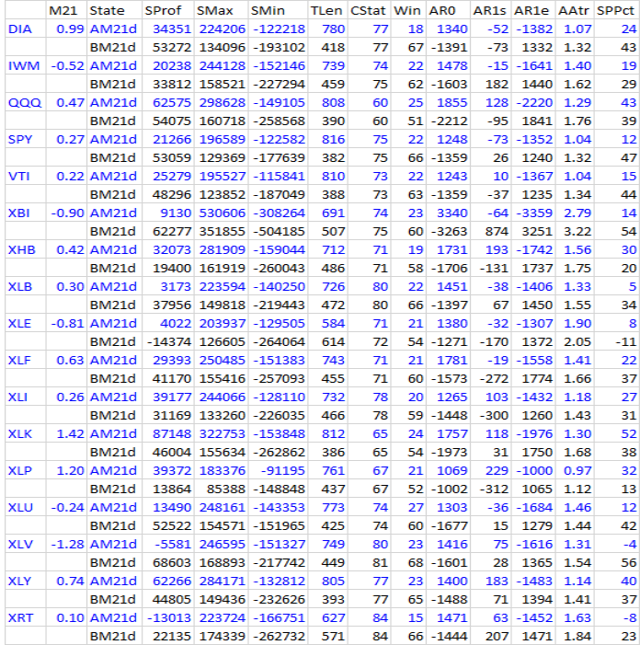Created by author using source data from Tiingo

This shows similar characteristics to the other time frames. Note, XLE was the only instrument to lose money in the below state, while XLV and XRT have losses in the above state. XLE is also notable because the TLen for below is greater than TLen for above. The M21d levels in the second column seems to reflect the near term current market environment pretty well.

## Conclusion

I have been working on this development effort since late last year. The tie in with my previous articles began to interest me a few weeks ago and writing this seemed like a good way to document the application.

Logan's article was an inspiration to me and I don't believe I could have developed this without the exchange of ideas with him.

Disclosure: I/we have no positions in any stocks mentioned, and no plans to initiate any positions within the next 72 hours. I wrote this article myself, and it expresses my own opinions. I am not receiving compensation for it. I have no business relationship with any company whose stock is mentioned in this article.